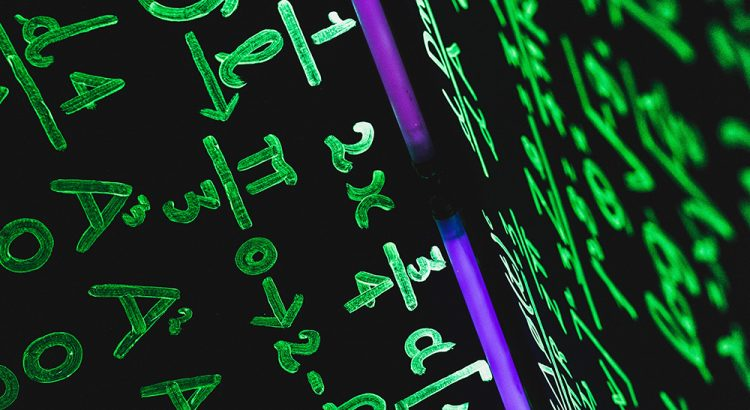# Mathematical Physicist

A mathematical physicist applies mathematics to problems in physics and develops mathematical methods suitable for the formulation of physical theories.

 Low End Salary:   \$55,610/yrMedian Salary:   \$111,580/yrHigh End Salary:   \$185,230/yr## Education:

Usually a Ph.D. in mathematics or physics is needed to work as a mathematical physicist. People who want permanent jobs in basic research at universities and government labs need even more training. People with a master's degree in physics often get jobs in manufacturing and applied research and development. Those with a bachelor's degree often work as technicians or research assistants.

## Math Required:

College Algebra, Trigonometry, Calculus I and II, Linear Algebra, Differential Equations, Topology, Combinatorics

## When Math is Used:

Math is constantly used as a mathematical physicist as they use models and equations to solve a variety of physics-related problems. Mathematical physics refers to development of mathematical methods for application to problems in physics. The Journal of Mathematical Physics defines the field as: "the application of mathematics to problems in physics and the development of mathematical methods suitable for such applications and for the formulation of physical theories."

## Potential Employers:

About 38 percent of mathematical physicists work in scientific research and development services firms. Another 21 percent work for the Federal Government, mostly in the Department of Defense or the National Aeronautics and Space Administration. Others work in colleges and universities, usually in research.

## Facts:

• Sir Isaac Newton, the great English physicist and mathematician, developed a wealth of new mathematics to solve problems in physics • Most mathematical physicists will spend their time researching ideas that will help further our understanding of how things work

## Citations:

• http://en.wikipedia.org/wiki/Mathematical_physics
• http://www.bls.gov/oes/current/oes192012.htm